# Tag: cryptography

One of the trends of 2010 was the proliferation of StackExchange sites. I guess by now most of us visit MathOverflow along with the arXiv daily. But, there are plenty of other StackExchange sites around that may be of interest to the mathematics-community :

“Opening a StackExchange site is damn hard. First you have to find at least 60 people interested in the site. Then, when this limit is reached, a large amount of people (in the hundreds, but it really depends on the reputation of each participant) must commit and promise to create momentum for the site, adding questions and answers. When this amount is reached, the site is open and stays in closed beta for seven days. During this time, the committers have to enrich the site so that the public beta (which starts after the first seven days) gets enough hits and participants to show a self-sustained community.” (quote from ForTheScience’s StackExchange sites proliferation, this post also contains a list of StackExchange-projects in almost every corner of Life)

The site keeping you up to date with StackExchange proposals and their progress is area51. Perhaps, you want to commit to some of these proposals

or simply browse around area51 until you find the ideal community for you to belong to…

In ONAG, John Conway proves that the symmetric version of his recursive definition of addition and multiplcation on the surreal numbers make the class On of all Cantor’s ordinal numbers into an algebraically closed Field of characteristic two : On2 (pronounced ‘Onto’), and, in particular, he identifies a subfield
with the algebraic closure of the field of two elements. What makes all of this somewhat confusing is that Cantor had already defined a (badly behaving) addition, multiplication and exponentiation on ordinal numbers.

Over the last week I’ve been playing a bit with sage to prove a few exotic identities involving ordinal numbers. Here’s one of them ($\omega$ is the first infinite ordinal number, that is, $\omega={ 0,1,2,\ldots }$),

$~(\omega^{\omega^{13}})^{47} = \omega^{\omega^7} + 1$

answering a question in Hendrik Lenstra’s paper Nim multiplication.

However, it will take us a couple of posts before we get there. Let’s begin by trying to explain what brought this on. On september 24th 2008 there was a meeting, intended for a general public, called a la rencontre des dechiffeurs, celebrating the 50th birthday of the IHES.

One of the speakers was Alain Connes and the official title of his talk was “L’ange de la géométrie, le diable de l’algèbre et le corps à un élément” (the angel of geometry, the devil of algebra and the field with one element). Instead, he talked about a seemingly trivial problem : what is the algebraic closure of $\mathbb{F}_2$, the field with two elements? My only information about the actual content of the talk comes from the following YouTube-blurb

Alain argues that we do not have a satisfactory description of $\overline{\mathbb{F}}_2$, the algebraic closure of $\mathbb{F}_2$. Naturally, it is the union (or rather, limit) of all finite fields $\mathbb{F}_{2^n}$, but, there are too many non-canonical choices to make here.

Recall that $\mathbb{F}_{2^k}$ is a subfield of $\mathbb{F}_{2^l}$ if and only if $k$ is a divisor of $l$ and so we would have to take the direct limit over the integers with respect to the divisibility relation… Of course, we can replace this by an increasing sequence of a selection of cofinal fields such as

$\mathbb{F}_{2^{1!}} \subset \mathbb{F}_{2^{2!}} \subset \mathbb{F}_{2^{3!}} \subset \ldots$

But then, there are several such suitable sequences! Another ambiguity comes from the description of $\mathbb{F}_{2^n}$. Clearly it is of the form $\mathbb{F}_2[x]/(f(x))$ where $f(x)$ is a monic irreducible polynomial of degree $n$, but again, there are several such polynomials. An attempt to make a canonical choice of polynomial is to take the ‘first’ suitable one with respect to some natural ordering on the polynomials. This leads to the so called Conway polynomials.

Conway polynomials for the prime $2$ have only been determined up to degree 400-something, so in the increasing sequence above we would already be stuck at the sixth term $\mathbb{F}_{2^{6!}}$…

So, what Alain Connes sets as a problem is to find another, more canonical, description of $\overline{\mathbb{F}}_2$. The problem is not without real-life interest as most finite fields appearing in cryptography or coding theory are subfields of $\overline{\mathbb{F}}_2$.

(My guess is that Alain originally wanted to talk about the action of the Galois group on the roots of unity, which would be the corresponding problem over the field with one element and would explain the title of the talk, but decided against it. If anyone knows what ‘coupling-problem’ he is referring to, please drop a comment.)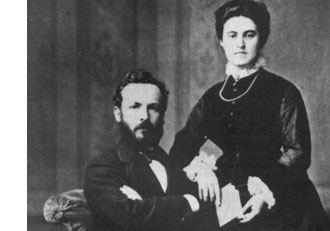Surely, Connes is aware of the fact that there exists a nice canonical recursive construction of $\overline{\mathbb{F}}_2$ due to John Conway, using Georg Cantor’s ordinal numbers.

In fact, in chapter 6 of his book On Numbers And Games, John Conway proves that the symmetric version of his recursive definition of addition and multiplcation on the surreal numbers make the class $\mathbf{On}$ of all Cantor’s ordinal numbers into an algebraically closed Field of characteristic two : $\mathbf{On}_2$ (pronounced ‘Onto’), and, in particular, he identifies a subfield

$\overline{\mathbb{F}}_2 \simeq [ \omega^{\omega^{\omega}} ]$

with the algebraic closure of $\mathbb{F}_2$. What makes all of this somewhat confusing is that Cantor had already defined a (badly behaving) addition, multiplication and exponentiation on ordinal numbers. To distinguish between the Cantor/Conway arithmetics, Conway (and later Lenstra) adopt the convention that any expression between square brackets refers to Cantor-arithmetic and un-squared ones to Conway’s. So, in the description of the algebraic closure just given $[ \omega^{\omega^{\omega}} ]$ is the ordinal defined by Cantor-exponentiation, whereas the exotic identity we started out with refers to Conway’s arithmetic on ordinal numbers.

Let’s recall briefly Cantor’s ordinal arithmetic. An ordinal number $\alpha$ is the order-type of a totally ordered set, that is, if there is an order preserving bijection between two totally ordered sets then they have the same ordinal number (or you might view $\alpha$ itself as a totally ordered set, namely the set of all strictly smaller ordinal numbers, so e.g. $0= \emptyset,1= { 0 },2={ 0,1 },\ldots$).

For two ordinals $\alpha$ and $\beta$, the addition $[\alpha + \beta ]$ is the order-type of the totally ordered set $\alpha \sqcup \beta$ (the disjoint union) ordered compatible with the total orders in $\alpha$ and $\beta$ and such that every element of $\beta$ is strictly greater than any element from $\alpha$. Observe that this definition depends on the order of the two factors. For example,$[1 + \omega] = \omega$ as there is an order preserving bijection ${ \tilde{0},0,1,2,\ldots } \rightarrow { 0,1,2,3,\ldots }$ by $\tilde{0} \mapsto 0,n \mapsto n+1$. However, $\omega \not= [\omega + 1]$ as there can be no order preserving bijection ${ 0,1,2,\ldots } \rightarrow { 0,1,2,\ldots,0_{max} }$ as the first set has no maximal element whereas the second one does. So, Cantor’s addition has the bad property that it may be that $[\alpha + \beta] \not= [\beta + \alpha]$.

The Cantor-multiplication $\alpha . \beta$ is the order-type of the product-set $\alpha \times \beta$ ordered via the last differing coordinate. Again, this product has the bad property that it may happen that $[\alpha . \beta] \not= [\beta . \alpha]$ (for example $[2 . \omega ] \not=[ \omega . 2 ]$). Finally, the exponential $\beta^{\alpha}$ is the order type of the set of all maps $f~:~\alpha \rightarrow \beta$ such that $f(a) \not=0$ for only finitely many $a \in \alpha$, and ordered via the last differing function-value.

Cantor’s arithmetic allows normal-forms for ordinal numbers. More precisely, with respect to any ordinal number $\gamma \geq 2$, every ordinal number $\alpha \geq 1$ has a unique expression as

$\alpha = [ \gamma^{\alpha_0}.\eta_0 + \gamma^{\alpha_1}.\eta_1 + \ldots + \gamma^{\alpha_m}.\eta_m]$

for some natural number $m$ and such that $\alpha \geq \alpha_0 > \alpha_1 > \ldots > \alpha_m \geq 0$ and all $1 \leq \eta_i < \gamma$. In particular, taking the special cases $\gamma = 2$ and $\gamma = \omega$, we have the following two canonical forms for any ordinal number $\alpha$

$[ 2^{\alpha_0} + 2^{\alpha_1} + \ldots + 2^{\alpha_m}] = \alpha = [ \omega^{\beta_0}.n_0 + \omega^{\beta_1}.n_1 + \ldots + \omega^{\beta_k}.n_k]$

with $m,k,n_i$ natural numbers and $\alpha \geq \alpha_0 > \alpha_1 > \ldots > \alpha_m \geq 0$ and $\alpha \geq \beta_0 > \beta_1 > \ldots > \beta_k \geq 0$. Both canonical forms will be important when we consider the (better behaved) Conway-arithmetic on $\mathbf{On}_2$, next time.

I couldn’t believe my eyes. I was watching an episode of numb3rs, ‘undercurrents’ to be precise, and there it was, circled in the middle of the blackboard, CEILIDH, together with some of the key-exchange maps around it…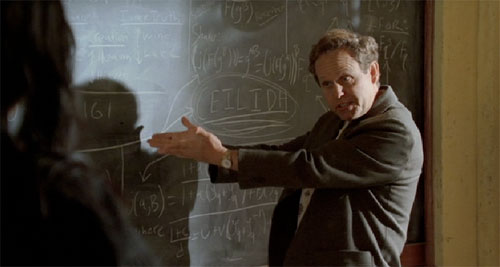Only, the plot doesn’t involve any tori-crypto… okay, there is an I-Ching-coded-tattoo which turns out to be a telephone number, but that’s all. Still, this couldn’t just be a coincidence. Googling for ‘ceilidh+numbers‘ gives as top hit the pdf-file of an article Alice in NUMB3Rland written by … Alice Silverberg (of the Rubin-Silverberg paper starting tori-cryptography). Alice turns out to be one of the unpaid consultants to the series. The 2-page article gives some insight into how ‘some math’ gets into the script

Typically, Andy emails a draft of the
script to the consultants. The FBI plot
is already in place, and the writers want
mathematics to go with it. The placeholder “math” in the draft is often nonsense or
jargon; the sort of things people with no
mathematical background might find by
Googling, and think was real math. Since
there’s often no mathematics that makes
sense in those parts of the script, the best
the consultants can do is replace jargon
that makes us cringe a lot with jargon that
makes us cringe a little.

From then on, it’s the Telephone Game.
The consultants email Andy our suggestions (“replace ‘our discrete universes’
with ‘our disjoint universes'”; “replace
the nonsensical ‘we’ve tried everything
-a full frequency analysis, a Vignere
deconstruction- we even checked for
a Lucas sequence’ with the slightly less
nonsensical ‘It’s much too short to try
any cryptanalysis on. If it were longer
we could try frequency analyses, or try
to guess what kind of cryptosystem it is
and use a specialized technique. For example, if it were a long enough Vigenere
cipher we could try a Kasiski test or an
index-of-coincidence analysis’). Andy
chooses about a quarter of my sugges-
tions and forwards his interpretation
of them to the writers and producers.
The script gets changed, and then the
actors ad lib something completely dif-
ferent (‘disjointed universes’: cute, but
loses the mathematical allusion; ‘Kasiski
exam’ : I didn’t mean that kind of ‘test’).

She ends her article with :

I have mixed feelings about NUMB3RS. I still have concerns about the violence, the depiction of women, and the pretense
that the math is accurate. However, if NUMB3RS could interest people in the power of mathematics enough for society
to greater value and support mathematics teaching, learning, and research, and
motivate more students to learnthat would be a positive step.

Further, there is a whole blog dedicated to some of the maths featuring in NUMB3RS, the numb3rs blog. And it was the first time I had to take a screenshot of a DVD, something usually off limits to the grab.app, but there is a simple hack to do it…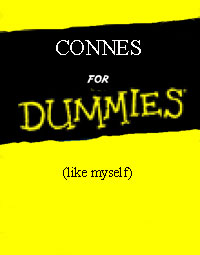You may not have noticed, but the really hard work was done behind the scenes, resurrecting about 300 old posts (some of them hidden by giving them ‘private’-status). Ive only deleted about 10 posts with little or no content and am sorry I’ve self-destructed about 20-30 hectic posts over the years by pressing the ‘delete post’ button. I would have liked to reread them after all the angry mails Ive received. But, as Ive defended myself at the time, and as I continue to do today, a blog only records feelings at a specific moment. Often, the issue is closed for me once Ive put my frustrations in a post, and then Ill forget all about it. Sadly, the gossip-circuit in noncommutative circles is a lot, a lot, slower than my mood swings, so by the time people complain it’s no longer an issue for me and I tend to delete the post altogether. A blog really is a sort of diary. For example, it only struck me now, rereading the posts of the end of 2006, beginning of 2007, how depressed I must have been at the time. Fortunately, life has improved, somewhat… Still, after all these reminiscences, the real issue is : what comes next?

Some of you may have noticed that I’ve closed the open series on tori-cryptography and on superpotentials in a rather abrupt manner. It took me that long to realize that none of you is waiting for this kind of posts. You’re thinking : if he really wants to show off, let him do his damned thing on the arXiv, a couple of days a year, at worst, and then we can then safely ignore it, like we do with most papers. Isnt’t that true? Of course it is…

So, what are you waiting for? Here’s what I believe to be a sensible thing to try out. Over the last 4 years I must have posted well over 50 times what I believe noncommutative geometry is all about, so if you still don’t know, please consult the archive, I fear I can only repeat myself. Probably, it is more worthwhile to reach out to other approaches to noncommutative geometry, trying to figure out what, if anything, they are after, without becoming a new-age convert (‘connes-vert’, I’d say). The top-left picture may give you an inkling of what I’m after… Besides, Im supposed to run a ‘capita selecta’ course for third year Bachelors and Ive chosen to read with them the book The music of the primes and to expand on the mathematics hinted only at in the book. So, I’ll totally immerse myself in Connes’ project to solve the Riemann-hypothesis in the upcoming months.

Again, rereading old posts, it strikes me how much effort I’ve put into trying to check whether technology can genuinely help mathematicians to do what they want to do more efficiently (all post categorized as iMath). I plan some series of posts re-exploring these ideas. The first series will be about the overhyped Web-2 thing of social-bookmarking. So, in the next weeks I’ll go undercover and check out which socialsites are best for mathematicians (in particular, noncommutative geometers) to embrace…

Apart from these, admittedly vague, plans I am as always open for suggestions you might have. So, please drop a comment..

Last time we have seen that tori are dual (via their group of characters) to lattices with a Galois action. In particular, the Weil descent torus $R_n=R^1_{\mathbb{F}_{p^n}/\mathbb{F}_p} \mathbb{G}_m$ corresponds to the permutation lattices $R_n^* = \mathbb{Z}[x]/(x^n-1)$. The action of the generator $\sigma$ (the Frobenius) of the Galois group $Gal(\mathbb{F}_{p^n}/\mathbb{F}_p)$ acts on the lattice by multiplication with $x$.

An old result of Masuda (1955), using an even older lemma by Speiser (1919), asserts than whenever the character-lattice $T^*$ of a torus $T$ is a permutation-lattice, the torus is rational, that is, the function-field
of the torus $\mathbb{F}_p(T)$ is purely trancendental

$\mathbb{F}_p(y_1,\ldots,y_d) = \mathbb{F}_p(T) = (\mathbb{F}_{q^n}(T^*))^{Gal}$

(recall from last time that the field on the right-hand side is the field of fractions of the $Gal$-invariants of the group-algebra of the free Abelian group $T^* = \mathbb{Z} \oplus \ldots \oplus \mathbb{Z}$ where the rank is equal to the dimension $d$ of the torus).

The basic observation made by Rubin and Silverberg was that the known results on crypto-compression could be reformulated in the language of algebraic tori as : the tori $T_2$ (LUC-system) and $T_6$ (CEILIDH-system) are rational! So, what about the next cryptographic challenges? Are the tori $T_{30}$, $T_{210}$ etc. also rational varieties?

Recall that as a group, the $\mathbb{F}_p$-points of the torus $T_n$, is the subgroup of $\mathbb{F}_{p^n}^*$ corresponding to the most crypto-challenging cyclic subgroup of order $\Phi_n(p)$ where $\Phi_n(x)$ is the n-th cyclotomic polynomial. The character-lattice of this crypto-torus $T_n$ we call the crypto-lattice and it is

$T_n^* = \mathbb{Z}[x]/(\Phi_n(x))$

(again the action of the Frobenius is given by multiplication with $x$) and hence has rank $\phi(n)$, explaining that the torus $T_n$ has dimension $\phi(n)$ and hence that we can at best expect a compression from $n$-pits to $\phi(n)$-pits. Note that the lattice $T_n^*$ is no longer a permutation lattice, so we cannot use the Masuda-Speiser result to prove rationality of $T_n$.

What have mathematicians proved on $T_n$ before it became a hot topic? Well, there is an old conjecture by V. E. Voskresenskii asserting that all $T_n$ should be rational! Unfortunately, he could prove this only when $n$ is a prime power. Further, he proved that for all $n$, the lattice $T_n$ is at least stably-rational meaning that it is rational upto adding free parameters, that is

$\mathbb{F}_p(T_n)(z_1,\ldots,z_l) = \mathbb{F}_p(y_1,\ldots,y_{d+l})$

which, sadly, is only of cryptographic-use if $l$ is small (see below). A true rationality result on $T_n$ was proved by A.A. Klyashko : $T_n$ is rational whenever $n=p^a.q^b$ a product of two prime powers.But then, $30=2 \times 3 \times 5$ the first unknown case…

At Crypto 2004, Marten van Dijk and David Woodruff were able to use an explicit form of Voskresenskii stable rationality result to get an asymptotic optimal crypto-compression rate of $n/\phi(n)$, but their method was of little practical use in the $T_{30}$, for what their method gave was a rational map

$T_{30} \times \mathbb{A}^{32}_{\mathbb{F}_p} \rightarrow \mathbb{A}^{40}_{\mathbb{F}_p}$

and the number of added parameters (32) is way too big to be of use.

But then, one can use century-old results on cyclotomic polynomials to get a much better bound, as was shown in the paper Practical cryptography in high dimensional tori by the collective group of all people working (openly) on tori-cryptography. The idea is that whenever q is a prime and a is an integer not divisible by q, then on the level of cyclotomic polynomials we have the identity

$\Phi_{aq}(x) \Phi_a(x) = \Phi_a(x^q)$

On the level of tori this equality implies (via the character-lattices) an ismorphism (with same assumptions)

$T_{aq}(\mathbb{F}_p) \times T_a(\mathbb{F}_p) \simeq (R^1_{\mathbb{F}_{p^q}/\mathbb{F}_p} T_a)(\mathbb{F}_p) = T_a(\mathbb{F}_{p^q})$

whenever aq is not divisible by p. Apply this to the special case when $q=5,a=6$ then we get

$T_{30}(\mathbb{F}_p) \times T_6(\mathbb{F}_p) \simeq R^1_{\mathbb{F}_{p^5}/\mathbb{F}_p} T_6(\mathbb{F}_p)$

and because we know that $T_6$ is a 2-dimensional rational torus we get, using Weil descent, a rational map

$T_{30} \times \mathbb{A}^2_{\mathbb{F}_p} \rightarrow \mathbb{A}^{10}_{\mathbb{F}_p}$

which can be used to get better crypto-compression than the CEILIDH-system!

This concludes what I know of the OPEN state of affairs in tori-cryptography. I’m sure ‘people in hiding’ know a lot more at the moment and, if not, I have a couple of ideas I’d love to check out. So, when I seem to have disappeared, you know what happened…

A classic Andre Weil-tale is his narrow escape from being shot as a Russian spy

The war was a disaster for Weil who was a conscientious objector and so wished to avoid military service. He fled to Finland, to visit Rolf Nevanlinna, as soon as war was declared. This was an attempt to avoid being forced into the army, but it was not a simple matter to escape from the war in Europe at this time. Weil was arrested in Finland and when letters in Russian were found in his room (they were actually from Pontryagin describing mathematical research) things looked pretty black. One day Nevanlinna was told that they were about to execute Weil as a spy, and he was able to persuade the authorities to deport Weil instead.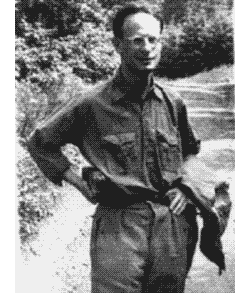However, Weil’s wikipedia entry calls this a story too good to be true, and continues

In 1992, the Finnish mathematician Osmo Pekonen went to the archives to check the facts. Based on the documents, he established that Weil was not really going to be shot, even if he was under arrest, and that Nevanlinna probably didn’t do – and didn’t need to do – anything to save him. Pekonen published a paper on this with an afterword by Andre Weil himself. Nevanlinna’s motivation for concocting such a story of himself as the rescuer of a famous Jewish mathematician probably was the fact that he had been a Nazi sympathizer during the war. The story also appears in Nevanlinna’s autobiography, published in Finnish, but the dates don’t match with real events at all. It is true, however, that Nevanlinna housed Weil in the summer of 1939 at his summer residence Korkee at Lohja in Finland – and offered Hitler’s Mein Kampf as bedside reading.

This old spy-story gets a recent twist now that it turns out that Weil’s descent theory of tori has applications to cryptography. So far, I haven’t really defined what tori are, so let us start with some basics.

The simplest (and archetypical) example of an algebraic torus is the multiplicative group(scheme) $\mathbb{G}_m$ over a finite field $\mathbb{F}_q$ which is the affine variety

$\mathbb{V}(xy-1) \subset \mathbb{A}^2_{\mathbb{F}_q}$. that is, the $\mathbb{F}_q$ points of $\mathbb{G}_m$ are precisely the couples ${ (x,\frac{1}{x})~:~x \in \mathbb{F}_q^* }$ and so are in one-to-one correspondence with the non-zero elements of $\mathbb{F}_q$. The coordinate ring of this variety is the ring of Laurant polynomials $\mathbb{F}_q[x,x^{-1}]$ and the fact that multiplication induces a group-structure on the points of the variety can be rephrased by saying that this coordinate ring is a Hopf algebra which is just the Hopf structure on the group-algebra $\mathbb{F}_q[\mathbb{Z}] = \mathbb{F}_q[x,x^{-1}]$. This is the first indication of a connection between tori defined over $\mathbb{F}_q$ and lattices (that is free $\mathbb{Z}$-modules with an action of the Galois group $Gal(\overline{F}_q/F_q)$. In this correspondence, the multiplicative group scheme $\mathbb{G}_m$ corresponds to $\mathbb{Z}$ with the trivial action.

Now take a field extension $\mathbb{F}_q \subset \mathbb{F}_{q^n}$, is there an affine variety, defined over $\mathbb{F}_q$ whose $\mathbb{F}_q$-points are precisely the invertible elements $\mathbb{F}_{q^n}^*$? Sure! Just take the multiplicative group over $\mathbb{F}_{q^n}$ and write the elements x and y as $x = x_1 + x_2 a_2 + \ldots + x_n a_n$ (and a similar expression for y with ${ 1,a_2,\ldots,a_n }$ being a basis of $\mathbb{F}_{q^n}/\mathbb{F}_q$ and write the defning equation $xy-1$ out, also with respect to this basis and this will then give you the equations of the desired variety, which is usually denoted by $R^1_{\mathbb{F}_{q^n}/\mathbb{F}_q} \mathbb{G}_m$ and called the Weil restriction of scalars torus.

A concrete example? Take $\mathbb{F}_9 = \mathbb{F}_3(\sqrt{-1})$ and write $x=x_1+x_2 \sqrt{-1}$ and $y=y_1+y_2 \sqrt{-1}$, then the defining equation $xy-1$ becomes

$~(x_1y_1-x_2y_2) + (x_1y_2-x_2y_1) \sqrt{-1} = 1$

whence $R^1_{\mathbb{F}_9/\mathbb{F}_3} = \mathbb{V}(x_1y_1-x_2y_2-1,x_1y_2-x_2y_1) \subset \mathbb{A}^4_{\mathbb{F}_3}$, the intersection of two quadratic hypersurfaces in 4-dimensional space.

Why do we call $R^1 \mathbb{G}_m$ a _torus_? Well, as with any variety defined over $\mathbb{F}_q$ we can also look at its points over a field-extension, for example over the algebraic closure $\overline{\mathbb{F}}_q$ and then it is easy to see that

$R^1_{\mathbb{F}_{q^n}/\mathbb{F}_q} \mathbb{G}_m (\overline{\mathbb{F}}_q) = \overline{\mathbb{F}}_q^* \times \ldots \times \overline{\mathbb{F}}_q^*$ (n copies)

and such algebraic groups are called tori. (To understand terminology, the compact group corresponding to $\mathbb{C}^* \times \mathbb{C}^*$ is $U_1 \times U_1 = S^1 \times S^1$, so a torus).

In fact, it is already the case that the $\mathbb{F}_{q^n}$ points of the restriction of scalar torus are $\mathbb{F}_{q^n}^* \times \ldots \times \mathbb{F}_{q^n}^*$ and therefore we call this field a splitting field of the torus.

This is the general definition of an algebraic torus : a torus T over $\mathbb{F}_q$ is an affine group scheme over $\mathbb{F}_q$ such that, if we extend scalars to the algebraic closure (and then it already holds for a finite extension) we get an isomorphism of affine group schemes

$T \times_{\mathbb{F}_q} \overline{\mathbb{F}}_q = \overline{\mathbb{F}}_q^* \times \ldots \times \overline{\mathbb{F}}_q^* = (\overline{\mathbb{F}}_q^*)^{n}$

in which case we call T a torus of dimension n. Clearly, the Galois group $Gal(\overline{\mathbb{F}}_q^*/\mathbb{F}_q)$ acts on the left hand side in such a way that we recover $T$ as the orbit space for this action.

Hence, anther way to phrase this is to say that an algebraic torus is the Weil descent of an action of the Galois group on the algebraic group $\overline{\mathbb{F}}_q^* \times \ldots \times \overline{\mathbb{F}}_q^*$.

Of course we can also rephrase this is more algebraic terms by looking at the coordinate rings. The coordinate ring of the algebraic group $~(\overline{\mathbb{F}}_q^*)^n$ is the group-algebra of the rank n lattice $\mathbb{Z}^n = \mathbb{Z} \oplus \ldots \oplus \mathbb{Z}$ (the free Abelian group of rank n), that is,
$\overline{\mathbb{F}}_q [ \mathbb{Z}^n ]$. Now the Galois group acts both on the field $\overline{\mathbb{F}}_q$ as on the lattice $\mathbb{Z}^n$ coming from the action of the Galois group on the extended torus $T \times_{\mathbb{F}_q} \overline{\mathbb{F}}_q$. In fact, it is best to denote this specific action on $\mathbb{Z}^n$ by $T^*$ and call $T^*$ the character group of $T$. Now, we recover the coordinate ring of the $\mathbb{F}_q$-torus $T$ as the ring of invariants

$\mathbb{F}_q[T] = \overline{\mathbb{F}}_q [T^*]^{Gal(\overline{\mathbb{F}}_q/\mathbb{F}_q)}$

Hence, the restriction of scalars torus $R^1_{\mathbb{F}_{q^n}/\mathbb{F}_q} \mathbb{G}_m$ is an n-dimensional torus over $\mathbb{F}_q$ and its corresponding character group is the free Abelian group of rank n which can be written as $\mathbb{Z}[x]/(x^n-1) = \mathbb{Z}1 \oplus \mathbb{Z}x \oplus \ldots \oplus \mathbb{Z}x^{n-1}$ and where the action of the cyclic Galois group $Gal(\mathbb{F}_{q^n}/\mathbb{F}_q) = C_n = \langle \sigma \rangle$ s such that the generator $\sigma$ as as multiplication by $x$. That is, in this case the character group is a permutation lattice meaning that the $\mathbb{Z}$-module has a basis which is permuted under the action of the Galois group. Next time we will encounter more difficult tori sich as the crypto-torus $T_n$.

The main application of tori to cryptography is to exchange keys more efficiently while preserving the same security standards.

In the Diffie-Hellman key-exchange one interchanges elements of the finite field $\mathbb{F}_q$ where $q=p^N$ is a prime-power of a large prime number $p$. If we call an element of the prime field $\mathbb{F}_p$ a pit (similar to bit when $p=2$) then we can measure transmssions in pits. An element $h \in \mathbb{F}_q$ requires N pits, for we can write the finite field as the quotient of ring of polynomials $\mathbb{F}_p[x]$

$\mathbb{F}_q = \frac{\mathbb{F}_p[x]}{(f(x))}$

modulo an _irreducible_ polynomial $f(x)$ of degree N. Hence, any $h \in \mathbb{F}_q$ can be written as a polynomial of degree $< N$,
$h = a_1 + a_2 x + \ldots + a_N x^{N-1}$
with all $a_i \in \mathbb{F}_p$, so we can represent $h=(a_1,a_2,\ldots,a_N)$ as N pits. Now, we are going to limit this number of pits (from $N$ to about $\phi(N)$ where $\phi$ is the Euler totient function, that is the number of integers smaller than N and coprime to it) by restricting the elements $h$ to be transfered to a subgroup of the group of units of the finite field $\mathbb{F}_q^*$ while not compromising on the security of the public key system (the large order of the basic element $g \in \mathbb{F}_q^*$ of which $h$ is a power).To see that this is indeed possible, let us consider the easiest case (that of $N=2$) and keep the discussion tori-free (those of you who know more will realize that Hilbert’s Satz 90 is never too far away…). If $q=p^2$ then the order of the cyclic group $\mathbb{F}_q^*$ is $p^2-1 = (p-1)(p+1)$ so in order to get a safe system let us choose the large prime number $p$ such that also tex/2=r $is a prime number. Right, now define$T_2 $to be the subgroup of$\mathbb{F}_q^* $of order$p+1 $and let$g $be a generator of it that we will use in the Diffie-Hellman exchange. Can we describe the element of$T_2 $(our torus in disguise)? Take$d \in \mathbb{F}_p^* $a non-square element, then we can write$\mathbb{F}_q = \mathbb{F}_p(\sqrt{d}) $and$T_2 = { a+b\sqrt{d}~:~(a+b\sqrt{d})^{p+1}=1 } $(here,$a,b \in \mathbb{F}_p $). But we claim that$~(a+b\sqrt{d})^p = a -b \sqrt{d} $. Indeed,$a^p=a,b^p=b $and from Fermat’s little theorem we deduce that$ -1 = (\frac{d}{p}) \equiv d^{\frac{p-1}{2}}~mod(p) $where the middle term is the Legendre symbol which is equal to -1 because d was a non-square modulo p. That is, we can then write$T_2 $as the algebraic variety of dimension one defined over$\mathbb{F}_p $and given by the equation$T_2 = { a+b\sqrt{d} \in \mathbb{F}_q^*~\mid~(a,b) \in \mathbb{F}^2~:~a^2-db^2=1 } $Because$T_2 $is of dimension one over$\mathbb{F}_p $we can hope that most of its elements can be represented by just one pit (instead of the two pits necessary to represent them as elements of$\mathbb{F}_q $). This is indeed the case, for we have explicit maps (in geometric terms, these maps show that$T_2 $is a rational variety)$j~:~\mathbb{F}_p \rightarrow T_2~\quad~j(a) = \frac{a+\sqrt{d}}{a-\sqrt{d}}=\frac{a^2+d}{a^2-d}+\frac{2a}{a^2-d}\sqrt{d} $which has a well-defined invers on the complement of${ 1,-1 } f~:~T_2 – { 1,-1 } \rightarrow \mathbb{F}_p~\quad~f(a+b\sqrt{d}) = \frac{1+a}{b} $From the right-hand description of$j(a) $one deduces that indeed we have that$f(j(a))=a $. Using this we can indeed compress the Diffie-Hellman exchange by a factor 2. Instead of giving you the element$g^a \in T_2 $computed using my secret number a, I’ll send you (using only one pit) the number$f(g^a) \in \mathbb{F}_p $. On this number, you can apply the j-function to recover$g^a $and then compute the common key$~(g^a)^b = g^{ab} $using your secret number b). Still, we didnt compromise on security because we used the most difficult elements around in$\mathbb{F}_q^* $. By going to higher dimensional tori one can even improve on the efficiency rate! Boris Kunyavskii arXived the paper Algebraic tori – thirty years after dedicated to the 80th anniversary of V. E. Voskresenskii. The goal is to give an overview of results of V. E. Voskresenskii on arithmetic and birational properties of algebraic tori which culminated in his monograph “Algebraic Tori” published in Russian 30 years ago. As Ive worked on this stuff a long time ago I glanced through the paper and it contains a nice summary of the work of V.E. Voskresenskii, and later of Jean-Louis Colliot-Thelene, Jean-Jacques Sansuc and David Saltman. To my surprise I also made a guest-appearance and even seem to have a conjecture (??!!). Fortunately the ‘conjecture’ turned out to be correct as was proved by Nicole Lemire and Martin Lorenz. But a much bigger surprise (at least to me) is contained in the final section of the paper where applications of (stable) rationality of certain tori are given to primality testing and public key cryptography! In [GPS] the authors propose to use a similar idea of compression for using tori in an even more recent cryptographic protocol (so-called pairing-based cryptography). It is interesting to note that the efficiency (compression factor) of the above mentioned cryptosystems heavily depends on rationality of tori under consideration (more precisely, on an explicit rational parameterization of the underlying variety). As the tori used by Rubin and Silverberg are known to be stably rational, the seemingly abstract question on rationality of a given stably rational torus is moving to the area of applied mathematics. The first challenging problem here is to obtain an explicit rational parameterization of the 8-dimensional torus$T_{30} $, deïfined over a finite field k and splitting over its cyclic extension L of degree 30. This is a particular case of a problem posed by Voskresenskii [Vo77, Problem 5.12] 30 years ago. Let us hope that we will not have to wait another 30 years for answering this question on a degree 30 extension. That’s all it takes to get me seriously side-tracked… so the last couple of hours I’ve been reading up on this connection between tori and cryptography. I will spend a couple of posts on these beautiful results. The latest seems to be that, while rationality of$T_{30} $is still unknown, one can use an explicit stable-rationality description of it to get a better bound than the XTR-system (the system corresponding to the torus$T_{6} $) which in turn is better than the LUC-system (corresponding to$T_2 $), which is turn is twice as efficient as the Diffie-Hellman key exchange system… So let us start gently with the latter one…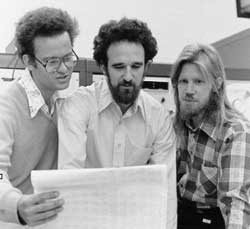Whitfield Diffie (r.) and Martin Hellman (m.) published in 1976 their public key-exchange system. Take a large prime power$q=p^N $, make it public and consider the finite field$\mathbb{F}_q $which is known to have a cyclic group of units$\mathbb{F}^*_q $of order$q-1 $. Now, take$g $to be an element in it of large order (preferable a generator but that isnt necessary) and also make this element public. Now choose a random integer$a $(your hidden secret) and compute the element$g^a \in \mathbb{F}_q $and publicize this element. Suppose someone else published his/her element$g^b $constructed from his/her secret integer$b $then both you and this other person can compute from the published data and their secret numbers the element (the shared key)$g^{ab}=(g^b)^a = (g^a)^b $(because you know$a $and the published$g^b $and your correspondent knows$b $and the published$g^a $) but nobody else can compute it from the public-available data only because discrete logarithms cannot be feasibly computed in the group$\mathbb{F}_q^* $. Hellman suggests to call this system the Diffie-Hellman-Merkl key-exchange (via this link) The first researchers to discover and publish the concepts of PKC were Whitfield Diffie and Martin Hellman from Stanford University, and Ralph Merkle from the University of California at Berkeley. As so often happens in the scientific world, the two groups were working independently on the same problem — Diffie and Hellman on public key cryptography and Merkle on public key distribution — when they became aware of each other’s work and realized there was synergy in their approaches. In Hellman’s words: “We each had a key part of the puzzle and while it’s true one of us first said X, and another of us first said Y, and so on, it was the combination and the back and forth between us that allowed the discovery.”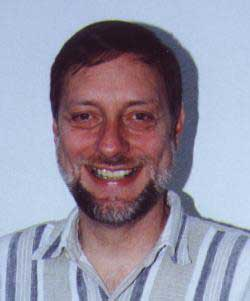And that was the full story until 1997. In December, 1997, it was revealed that researchers at the GCHQ organization did some work in the early 1970’s in the field of “non-secret encryption”. The people involved are James Ellis, Clifford Cocks and Malcolm Williamson (r.). Here is a note by Ellis on his recollection of the history of ‘Non-secret encryption” : Cryptography is a most unusual science. Most professional scientists aim to be the first to publish their work, because it is through dissemination that the work realises its value. In contrast, the fullest value of cryptography is realised by minimising the information available to potential adversaries. Thus professional cryptographers normally work in closed communities to provide sufficient professional interaction to ensure quality while maintaining secrecy from outsiders. Revelation of these secrets is normally only sanctioned in the interests of historical accuracy after it has been demonstrated clearly that no further benefit can be obtained from continued secrecy. In keeping with this tradition it is now appropriate to tell the story of the invention and development within CESG of non-secret encryption (NSE) which was our original name for what is now called PKC. The task of writing this paper has devolved on me because NSE was my idea and I can therefore describe these early developments from personal experience. No techniques not already public knowledge, or specific applications of NSE will be mentioned… The once secret notes of Williamson are also available. NON-SECRET ENCRYPTION USING A FINITE FIELD by M J Williamson, 21 January 1974 and THOUGHTS ON CHEAPER NON-SECRET ENCRYPTION M J Williamson, 10 August 1976 . A first year-first semester course on group theory has its hilarious moments. Whereas they can relate the two other pure math courses (linear algebra and analysis) _somewhat_ to what they’ve learned before, with group theory they appear to enter an entirely new and strange world. So, it is best to give them concrete examples : symmetry groups of regular polygons and Platonic solids, the symmetric group etc. One of the lesser traditional examples I like to give is Nim addition and its relation to combinatorial games. For their first test they had (among other things) to find a winning move for the position below in the Lenstra’s turtle turning game. At each move a player must put one turtle on its back and may also turn over any single turtle to the left of it. This second turtle, unlike the first, may be turned either onto its feet or onto its back. The player wins who turns the last turtle upside-down.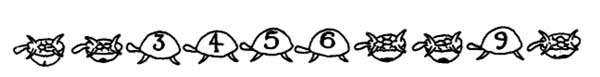So, all they needed to see was that one turtle on its feet at place n is equivalent to a Nim-heap of height n and use the fact that all elements have order two to show that any zero-move in the sum game can indeed be played by using the second-turtle alternative. (( for the curious : the answer is turning both 9 and 4 on their back )) A week later, one of the girls asked at the start of the lecture : Are there real-life applications of group-theory? I mean, my father asked me what I was learning at school and I told him we were playing games turning turtles. I have to say that he was not impressed at all!. She may have had an hidden agenda to slow me down because I spend an hour talking about a lot of things ranging from codes to cryptography and from representations to elementary particles… For test three (on group-actions) I asked them to prove (among other things) Wilson’s theorem that is$~(p-1)! \equiv -1~\text{mod}~p $for any prime number$p $. The hint being : take the trivial action of$S_p $on a one-element set and use the orbit theorem. (they know the number of elements in an$S_n $-conjugacy class) Fingers crossed, hopefully daddy approved… Suppose for a moment that some librarian at the Bodleian Library announces that (s)he discovered an old encrypted book attributed to Isaac Newton. After a few months of failed attempts, the code is finally cracked and turns out to use a Public Key system based on the product of two gigantic prime numbers,$2^{32582657}-1 $and$2^{30402457}-1 \$, which were only discovered to be prime recently. Would one deduce from this that Newton invented public key cryptography and that he used alchemy to factor integers? (( Come to think of it, some probably would ))

The cynic in me would argue that it is a hell of a coincidence for this text to surface exactly at the moment in history when we are able to show these numbers to be prime and understand their cryptographic use, and conclude that the book is likely to be a fabrication. Still, stranger things have happened in the history of mathematics…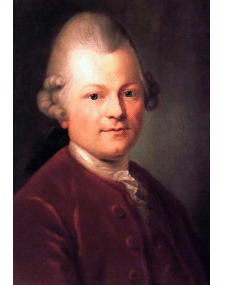In 1773, Gotthold Ephraim Lessing at that time librarian at the Herzog-August-Bibliothek discovered and published a Greek epigram in 22 elegiac couplets. The manuscript describes a problem sent by Archimedes to the mathematicians in Alexandria.

In his beautiful book “Number Theory, an approach through history. From Hammurapi to Legendre” Andre Weil asserts (( Chapter I,IX )):

Many mathematical epigrams are known. Most of them state problems of little depth; not so Lessing’s find; there is indeed every reason to accept the attribution to Archimedes, and none for putting it into doubt.

This Problema Bovidum (the cattle problem) is a surprisingly difficult diophantine problem and the simplest complete solution consists of eigth numbers, each having about 206545 digits. As we will see later the final ingredient in the solution is the solution of Pell’s equation using continued fractions discovered by Lagrange in 1768 and published in 1769 in a long memoir. Lagrange’s solution to the Pell equation was inserted in Euler’s “Algebra” which was composed in 1771 but published only in 1773… the very same year as Lessing’s discovery! (( all dates learned from Weil’s book Chp. III,XII ))

Weil’s book doesn’t include the details of the original epigram. The (lost) archeologist in me wanted to see the original Greek 22 couplets as well as a translation. So here they are : (( thanks to the Cattle problem site ))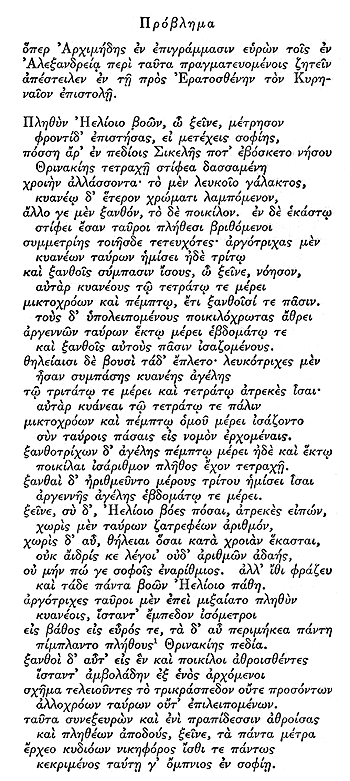A PROBLEM

which Archimedes solved in epigrams, and which he communicated to students of such matters at Alexandria in a letter to Eratosthenes of Cyrene.

If thou art diligent and wise, O stranger, compute the number of cattle of the Sun, who once upon a time grazed on the fields of the Thrinacian isle of Sicily, divided into four herds of different colours, one milk white, another a glossy black, a third yellow and the last dappled. In each herd were bulls, mighty in number according to these proportions: Understand, stranger, that the white bulls were equal to a half and a third of the black together with the whole of the yellow, while the black were equal to the fourth part of the dappled and a fifth, together with, once more, the whole of the yellow. Observe further that the remaining bulls, the dappled, were equal to a sixth part of the white and a seventh, together with all of the yellow. These were the proportions of the cows: The white were precisely equal to the third part and a fourth of the whole herd of the black; while the black were equal to the fourth part once more of the dappled and with it a fifth part, when all, including the bulls, went to pasture together. Now the dappled in four parts were equal in number to a fifth part and a sixth of the yellow herd. Finally the yellow were in number equal to a sixth part and a seventh of the white herd. If thou canst accurately tell, O stranger, the number of cattle of the Sun, giving separately the number of well-fed bulls and again the number of females according to each colour, thou wouldst not be called unskilled or ignorant of numbers, but not yet shalt thou be numbered among the wise.

But come, understand also all these conditions regarding the cattle of the Sun. When the white bulls mingled their number with the black, they stood firm, equal in depth and breadth, and the plains of Thrinacia, stretching far in all ways, were filled with their multitude. Again, when the yellow and the dappled bulls were gathered into one herd they stood in such a manner that their number, beginning from one, grew slowly greater till it completed a triangular figure, there being no bulls of other colours in their midst nor none of them lacking. If thou art able, O stranger, to find out all these things and gather them together in your mind, giving all the relations, thou shalt depart crowned with glory and knowing that thou hast been adjudged perfect in this species of wisdom.

The Lessing epigram may very well be an extremely laborious hoax but it is still worth spending a couple of posts on it. It gives us the opportunity to retell the amazing history of Pell’s problem rangingfrom the ancient Greeks and Indians, over Fermat and his correspondents, to Euler and Lagrange (with a couple of recent heroes entering the story). And, on top of this, the modular group is all the time just around the corner…